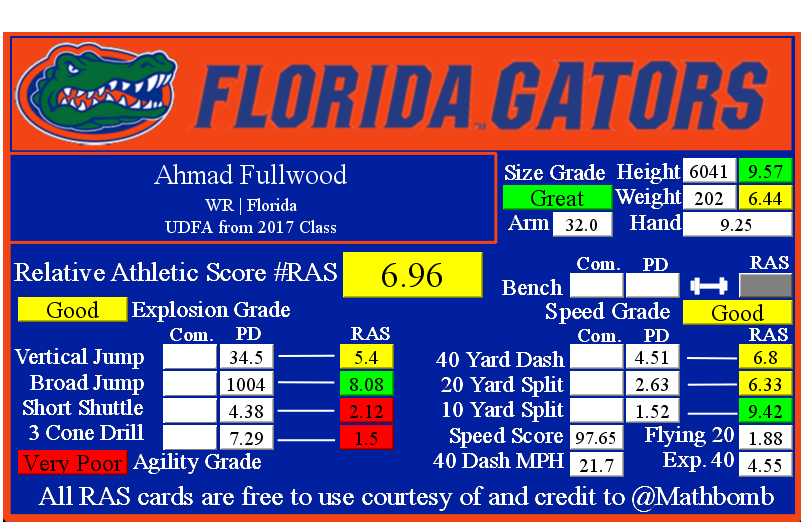Ahmad Fullwood went undrafted for the NFL in 2017.

He recorded a Relative Athletic Score of 6.96, out of a possible 10.0. RAS is a composite metric on a 0 to 10 scale based on the average of all of the percentile for each of the metrics the player completed either at the Combine or pro day.

He had a recorded height of 6041 that season, recorded as XYYZ where X is feet, YY is inches, and Z is eighths of an inch. That correlates to 6 feet, 4 and 1/8 of an inch or 76.125 inches, or 193.35750000000002 centimeters. This correlates to a 9.57 score out of 10.0.

He recorded a weight of 202 in pounds, which is approximately 91 kilograms. This correlates to a 6.44 score out of 10.0.

Based on his weight, he has a projected 40 yard dash time of 4.55. This is calculated by taking 0.00554 multiplied by his weight and then adding 3.433.
This player did not record any measurements at the NFL Combine that we were able to find.
This player did not have a recorded 40 yard dash for the Combine in the RAS database.

At his pro day, he recorded a 40 yard dash of 4.51 seconds. This correlates to a 6.8 score out of 10.0.

The time traveled between the 20 and 40 yard lines is known as the Flying Twenty. As the distance is also known, we can calculate the player’s speed over that distance. The time he traveled the last twenty yards at his pro day was 1.88 seconds. Over 20 yards, we can calculate his speed in yards per second to 10.64. Taking into account the distance in feet (60 feet), we can calculate his speed in feet per second to 31.91. Breaking it down further, we can calculate his speed in inches per second to 382.98. Knowing the feet per second of 31.91, we can calculate the approximate miles per hour by multiplying that value by 0.681818 to give us a calculated MPH of 21.8 in the last 20 yards of his run.

This player did not have a recorded 20 yard split for the Combine in the RAS database.

At his pro day, he recorded a 20 yard split of 2.63 seconds. This correlates to a 6.33 score out of 10.0.

We can calculate the speed traveled over the second ten yards of the 40 yard dash easily, as the distance and time are both known. The time he traveled the second ten yards at his pro day was 1.11 seconds. Over 10 yards, we can calculate his speed in yards per second to 9.01. Taking into account the distance in feet (30 feet), we can calculate his speed in feet per second to 27.03. Breaking it down further, we can calculate his speed in inches per second to 324.32. Knowing the feet per second of 27.03, we can calculate the approximate miles per hour by multiplying that value by 0.681818 to give us a calculated MPH of 18.4 in the second ten yards of his run.

This player did not have a recorded 10 yard split for the Combine in the RAS database.

At his pro day, he recorded a 10 yard split of 1.52 seconds. This correlates to a 9.42 score out of 10.0.

The time he traveled the first ten yards at his pro day was 1.52 seconds. Over 10 yards, we can calculate his speed in yards per second to 7.0. Taking into account the distance in feet (30 feet), we can calculate his speed in feet per second to 20.0. Breaking it down further, we can calculate his speed in inches per second to 237.0. Knowing the feet per second of 20.0, we can calculate the approximate miles per hour by multiplying that value by 0.681818 to give us a calculated MPH of 13.6 in the first ten yards of his run.

This player did not have a recorded bench press for the Combine in the RAS database.

This player did not have a recorded vertical jump for the Combine in the RAS database.

At his pro day, he recorded a vertical jump of 34.5 seconds. This correlates to a 5.4 score out of 10.0.

This player did not have a recorded broad jump for the Combine in the RAS database.

At his pro day, he recorded a broad jump of 1004, which is recorded as FII or FFII where F is feet and I is inches. This correlates to a 8.08 score out of 10.0.

This player did not have a recorded 5-10-5 or 20 yard short shuttle for the Combine in the RAS database.

At his pro day, he recorded a 5-10-5 or 20 yard short shuttle of 4.38 seconds. This correlates to a 2.12 score out of 10.0.

This player did not have a recorded 3 cone L drill for the Combine in the RAS database.

At his pro day, he recorded a 3 cone L drill of 7.29 seconds. This correlates to a 1.5 score out of 10.0.

This site uses Akismet to reduce spam. Learn how your comment data is processed.